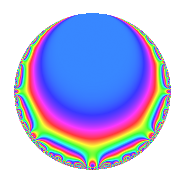# Properties

 Label 1155.2.a.pLevel 1155 Weight 2 Character orbit 1155.a Self dual Yes Analytic conductor 9.223 Analytic rank 0 Dimension 2 CM No Inner twists 1

# Learn more about

## Newspace parameters

 Level: $$N$$ = $$1155 = 3 \cdot 5 \cdot 7 \cdot 11$$ Weight: $$k$$ = $$2$$ Character orbit: $$[\chi]$$ = 1155.a (trivial)

## Newform invariants

 Self dual: Yes Analytic conductor: $$9.22272143346$$ Analytic rank: $$0$$ Dimension: $$2$$ Coefficient field: $$\Q(\sqrt{2})$$ Coefficient ring: $$\Z[a_1, a_2]$$ Coefficient ring index: $$1$$ Fricke sign: $$-1$$ Sato-Tate group: $\mathrm{SU}(2)$

## $q$-expansion

Coefficients of the $$q$$-expansion are expressed in terms of $$\beta = \sqrt{2}$$. We also show the integral $$q$$-expansion of the trace form.

 $$f(q)$$ $$=$$ $$q$$ $$+ \beta q^{2}$$ $$- q^{3}$$ $$- q^{5}$$ $$-\beta q^{6}$$ $$- q^{7}$$ $$-2 \beta q^{8}$$ $$+ q^{9}$$ $$+O(q^{10})$$ $$q$$ $$+ \beta q^{2}$$ $$- q^{3}$$ $$- q^{5}$$ $$-\beta q^{6}$$ $$- q^{7}$$ $$-2 \beta q^{8}$$ $$+ q^{9}$$ $$-\beta q^{10}$$ $$+ q^{11}$$ $$+ ( 2 + \beta ) q^{13}$$ $$-\beta q^{14}$$ $$+ q^{15}$$ $$-4 q^{16}$$ $$+ ( 1 + 2 \beta ) q^{17}$$ $$+ \beta q^{18}$$ $$+ ( -1 + 2 \beta ) q^{19}$$ $$+ q^{21}$$ $$+ \beta q^{22}$$ $$+ ( -1 + 2 \beta ) q^{23}$$ $$+ 2 \beta q^{24}$$ $$+ q^{25}$$ $$+ ( 2 + 2 \beta ) q^{26}$$ $$- q^{27}$$ $$+ ( 3 + 3 \beta ) q^{29}$$ $$+ \beta q^{30}$$ $$+ ( 2 + \beta ) q^{31}$$ $$- q^{33}$$ $$+ ( 4 + \beta ) q^{34}$$ $$+ q^{35}$$ $$+ ( -2 + 3 \beta ) q^{37}$$ $$+ ( 4 - \beta ) q^{38}$$ $$+ ( -2 - \beta ) q^{39}$$ $$+ 2 \beta q^{40}$$ $$+ ( 4 - 5 \beta ) q^{41}$$ $$+ \beta q^{42}$$ $$+ ( -5 + \beta ) q^{43}$$ $$- q^{45}$$ $$+ ( 4 - \beta ) q^{46}$$ $$+ ( 6 + 3 \beta ) q^{47}$$ $$+ 4 q^{48}$$ $$+ q^{49}$$ $$+ \beta q^{50}$$ $$+ ( -1 - 2 \beta ) q^{51}$$ $$+ ( 3 - 4 \beta ) q^{53}$$ $$-\beta q^{54}$$ $$- q^{55}$$ $$+ 2 \beta q^{56}$$ $$+ ( 1 - 2 \beta ) q^{57}$$ $$+ ( 6 + 3 \beta ) q^{58}$$ $$+ ( 13 - \beta ) q^{59}$$ $$+ ( 5 - 4 \beta ) q^{61}$$ $$+ ( 2 + 2 \beta ) q^{62}$$ $$- q^{63}$$ $$+ 8 q^{64}$$ $$+ ( -2 - \beta ) q^{65}$$ $$-\beta q^{66}$$ $$-6 q^{67}$$ $$+ ( 1 - 2 \beta ) q^{69}$$ $$+ \beta q^{70}$$ $$+ ( -4 - 5 \beta ) q^{71}$$ $$-2 \beta q^{72}$$ $$+ 6 \beta q^{73}$$ $$+ ( 6 - 2 \beta ) q^{74}$$ $$- q^{75}$$ $$- q^{77}$$ $$+ ( -2 - 2 \beta ) q^{78}$$ $$+ ( -4 + 3 \beta ) q^{79}$$ $$+ 4 q^{80}$$ $$+ q^{81}$$ $$+ ( -10 + 4 \beta ) q^{82}$$ $$+ ( 3 - 10 \beta ) q^{83}$$ $$+ ( -1 - 2 \beta ) q^{85}$$ $$+ ( 2 - 5 \beta ) q^{86}$$ $$+ ( -3 - 3 \beta ) q^{87}$$ $$-2 \beta q^{88}$$ $$+ ( 7 + 5 \beta ) q^{89}$$ $$-\beta q^{90}$$ $$+ ( -2 - \beta ) q^{91}$$ $$+ ( -2 - \beta ) q^{93}$$ $$+ ( 6 + 6 \beta ) q^{94}$$ $$+ ( 1 - 2 \beta ) q^{95}$$ $$+ ( -3 + 3 \beta ) q^{97}$$ $$+ \beta q^{98}$$ $$+ q^{99}$$ $$+O(q^{100})$$ $$\operatorname{Tr}(f)(q)$$ $$=$$ $$2q$$ $$\mathstrut -\mathstrut 2q^{3}$$ $$\mathstrut -\mathstrut 2q^{5}$$ $$\mathstrut -\mathstrut 2q^{7}$$ $$\mathstrut +\mathstrut 2q^{9}$$ $$\mathstrut +\mathstrut O(q^{10})$$ $$2q$$ $$\mathstrut -\mathstrut 2q^{3}$$ $$\mathstrut -\mathstrut 2q^{5}$$ $$\mathstrut -\mathstrut 2q^{7}$$ $$\mathstrut +\mathstrut 2q^{9}$$ $$\mathstrut +\mathstrut 2q^{11}$$ $$\mathstrut +\mathstrut 4q^{13}$$ $$\mathstrut +\mathstrut 2q^{15}$$ $$\mathstrut -\mathstrut 8q^{16}$$ $$\mathstrut +\mathstrut 2q^{17}$$ $$\mathstrut -\mathstrut 2q^{19}$$ $$\mathstrut +\mathstrut 2q^{21}$$ $$\mathstrut -\mathstrut 2q^{23}$$ $$\mathstrut +\mathstrut 2q^{25}$$ $$\mathstrut +\mathstrut 4q^{26}$$ $$\mathstrut -\mathstrut 2q^{27}$$ $$\mathstrut +\mathstrut 6q^{29}$$ $$\mathstrut +\mathstrut 4q^{31}$$ $$\mathstrut -\mathstrut 2q^{33}$$ $$\mathstrut +\mathstrut 8q^{34}$$ $$\mathstrut +\mathstrut 2q^{35}$$ $$\mathstrut -\mathstrut 4q^{37}$$ $$\mathstrut +\mathstrut 8q^{38}$$ $$\mathstrut -\mathstrut 4q^{39}$$ $$\mathstrut +\mathstrut 8q^{41}$$ $$\mathstrut -\mathstrut 10q^{43}$$ $$\mathstrut -\mathstrut 2q^{45}$$ $$\mathstrut +\mathstrut 8q^{46}$$ $$\mathstrut +\mathstrut 12q^{47}$$ $$\mathstrut +\mathstrut 8q^{48}$$ $$\mathstrut +\mathstrut 2q^{49}$$ $$\mathstrut -\mathstrut 2q^{51}$$ $$\mathstrut +\mathstrut 6q^{53}$$ $$\mathstrut -\mathstrut 2q^{55}$$ $$\mathstrut +\mathstrut 2q^{57}$$ $$\mathstrut +\mathstrut 12q^{58}$$ $$\mathstrut +\mathstrut 26q^{59}$$ $$\mathstrut +\mathstrut 10q^{61}$$ $$\mathstrut +\mathstrut 4q^{62}$$ $$\mathstrut -\mathstrut 2q^{63}$$ $$\mathstrut +\mathstrut 16q^{64}$$ $$\mathstrut -\mathstrut 4q^{65}$$ $$\mathstrut -\mathstrut 12q^{67}$$ $$\mathstrut +\mathstrut 2q^{69}$$ $$\mathstrut -\mathstrut 8q^{71}$$ $$\mathstrut +\mathstrut 12q^{74}$$ $$\mathstrut -\mathstrut 2q^{75}$$ $$\mathstrut -\mathstrut 2q^{77}$$ $$\mathstrut -\mathstrut 4q^{78}$$ $$\mathstrut -\mathstrut 8q^{79}$$ $$\mathstrut +\mathstrut 8q^{80}$$ $$\mathstrut +\mathstrut 2q^{81}$$ $$\mathstrut -\mathstrut 20q^{82}$$ $$\mathstrut +\mathstrut 6q^{83}$$ $$\mathstrut -\mathstrut 2q^{85}$$ $$\mathstrut +\mathstrut 4q^{86}$$ $$\mathstrut -\mathstrut 6q^{87}$$ $$\mathstrut +\mathstrut 14q^{89}$$ $$\mathstrut -\mathstrut 4q^{91}$$ $$\mathstrut -\mathstrut 4q^{93}$$ $$\mathstrut +\mathstrut 12q^{94}$$ $$\mathstrut +\mathstrut 2q^{95}$$ $$\mathstrut -\mathstrut 6q^{97}$$ $$\mathstrut +\mathstrut 2q^{99}$$ $$\mathstrut +\mathstrut O(q^{100})$$

## Embeddings

For each embedding $$\iota_m$$ of the coefficient field, the values $$\iota_m(a_n)$$ are shown below.

For more information on an embedded modular form you can click on its label.

Label $$\iota_m(\nu)$$ $$a_{2}$$ $$a_{3}$$ $$a_{4}$$ $$a_{5}$$ $$a_{6}$$ $$a_{7}$$ $$a_{8}$$ $$a_{9}$$ $$a_{10}$$
1.1
 −1.41421 1.41421
−1.41421 −1.00000 0 −1.00000 1.41421 −1.00000 2.82843 1.00000 1.41421
1.2 1.41421 −1.00000 0 −1.00000 −1.41421 −1.00000 −2.82843 1.00000 −1.41421
 $$n$$: e.g. 2-40 or 990-1000 Significant digits: Format: Complex embeddings Normalized embeddings Satake parameters Satake angles

## Inner twists

This newform does not admit any (nontrivial) inner twists.

## Atkin-Lehner signs

$$p$$ Sign
$$3$$ $$1$$
$$5$$ $$1$$
$$7$$ $$1$$
$$11$$ $$-1$$

## Hecke kernels

This newform can be constructed as the intersection of the kernels of the following linear operators acting on $$S_{2}^{\mathrm{new}}(\Gamma_0(1155))$$:

 $$T_{2}^{2}$$ $$\mathstrut -\mathstrut 2$$ $$T_{13}^{2}$$ $$\mathstrut -\mathstrut 4 T_{13}$$ $$\mathstrut +\mathstrut 2$$ $$T_{17}^{2}$$ $$\mathstrut -\mathstrut 2 T_{17}$$ $$\mathstrut -\mathstrut 7$$